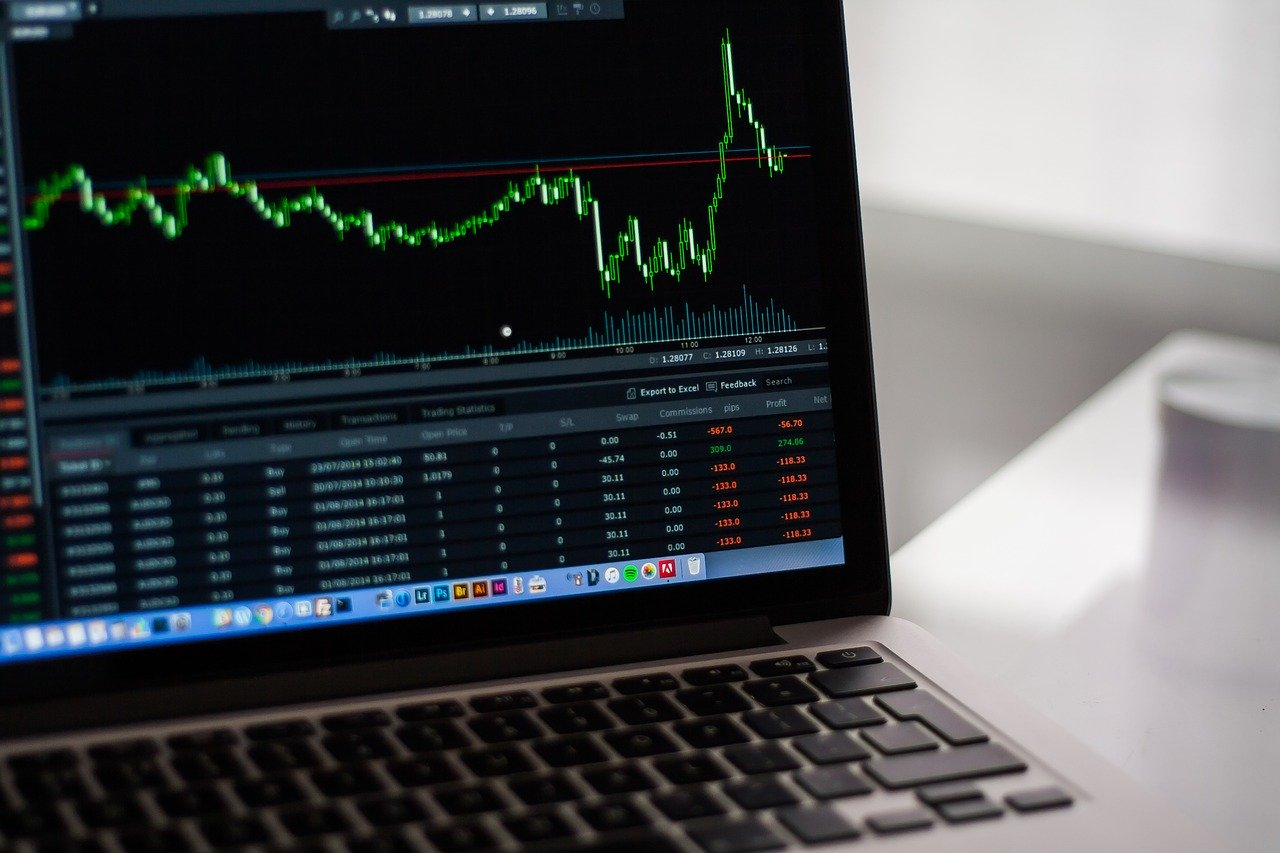# How to use the Exponential Moving Average in tradingThe Exponential Moving Average is a technical indicator that allows you to take a position on a financial asset with more flexibility.

In the Moving Average family, the Exponential Moving Average (or "EMA") assigns a regressive coefficient to the periods studied, so that more weight is given to recent fluctuations. The EMA is a tool to be used with care. Read on for essential information you need to know about this technical indicator and how to implement it effectively in your trading strategy.

## What is the Exponential Moving Average ?

The Exponential Moving Average is a type of Moving Average that aims to assign a coefficient whose value is proportional to the recentness of the period studied. Among the various types of Moving Averages used in technical analysis, the Exponential Moving Average (also abbreviated to EMA) is undoubtedly one of the most well-known. Indeed, its calculation formula allows it to give more weight to the recent periods of a price, compared to the older periods. The EMA thus offers greater flexibility, which is appreciated by traders seeking to detect financial market trends quickly.

To work with the Exponential Moving Average, you need to know two things :

• It requires the MME tool to be optimally configured, in particular by assigning a carefully chosen importance coefficient to each period.
• It is necessary to protect against false signals, which are more likely to occur when using the MME than when using a conventional moving average, since the most recent variations acquire more weight.

The Exponential Moving Average therefore places less importance on the underlying trend of a price. For this reason, it is preferable - as with any technical analysis tool - to use it in addition to other longer-term technical indicators.

The Exponential Moving Average plays a role in the analysis of many economic contexts and is generally used in the following ways by experienced traders :

• As a tool for confirming an already existing trend in financial prices
• As a trend reversal detection tool
• As a data smoothing tool, to gain visibility on trends

A good reading of the Exponential Moving Average allows you to identify the best time to take a position on a financial asset, both for buying and selling, provided that you avoid false signals.

## Calculation of the Exponential Moving Average

The Exponential Moving Average is composed of several elements that can be calculated beforehand :

• The Multiplier, expressed as a percentage and is equal to 2 divided by the number of periods taken into account plus 1. In other words, it can be expressed as follows: M = 2/(Number of Periods + 1)
• The Current Price, which shows the value of the studied asset at the current time N and can be expressed as Course[N]
• The Exponential Moving Average of the previous day, expressed as MME[N-1]

The formula for calculating the Exponential Moving Average is as follows : MME = MME[N-1] + M x (Price[N] - MME[N-1])

Unlike the SMA (Simple Moving Average), the Exponential Moving Average thus obtained will be expressed as a percentage and not as an absolute value.

Good to know : In the formula for calculating the M multiplier, you will see that the higher the number of periods taken into consideration, the lower it will be. It is therefore thanks to it that the oldest prices will have a relatively less significant value. Conversely, the most recent prices will gain weight in the interpretation of this technical indicator.

## Exponential, weighted or simple : which Moving Average should you choose ?

There are three main types of Moving Average.

### The Simple Moving Average (SMA)

The Simple Moving Average - also known as "Classic" - is preferred by novice investors seeking a simple and easy-to-read tool. Since it does not assign a coefficient to the periods studied, it is easier to interpret and offers fewer false buy and sell signals, but also less flexibility in the face of price volatility. Nevertheless, many experienced investors use it in their strategy in addition to other tools.

### The Weighted Moving Average (WMA)

The Weighted Moving Average, unlike the WMA, assigns a more refined value to the selected periods. However, it will not include the oldest periods : its information is therefore limited in time.

### The Exponential Moving Average (EMA)

The Exponential Moving Average remains the most common moving average on the financial markets. Because it requires a certain knowledge of technical analysis, it is preferable for beginners to start with the SMA and then implement the EMA in a demo account.

Note : Because these three technical indicators offer different but complementary functionalities, it is important to base your choice of tools on your investor profile, your trading strategy and your action plan.

## Conclusion

Although its calculation formula is more complex than other Moving Averages, the Exponential Moving Average offers a more intuitive and flexible reading of stock prices. Thus, studied with other technical indicators - to avoid false signals - it will allow the informed investor to take position on the reinforcement as on the reversal of the trend.